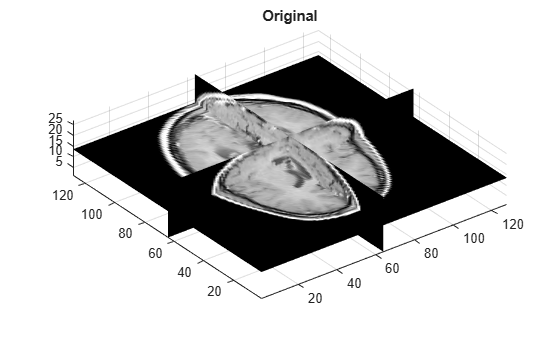# imresize3

Resize 3-D volumetric intensity image

## Syntax

``B = imresize3(V,scale)``
``````B = imresize3(V,[numrows numcols numplanes])``````
``B = imresize3(___,method)``
``B = imresize3(___,Name,Value)``

## Description

example

````B = imresize3(V,scale)` returns the volume `B` that is `scale` times the size of 3-D numeric or categorical volume `V`.```
``````B = imresize3(V,[numrows numcols numplanes])``` returns the volume `B` that has the number of rows, columns, and planes specified by the 3-element vector ```[numrows numcols numplanes]```.```
````B = imresize3(___,method)` returns the volume `B`, where `method` specifies the interpolation method used.```
````B = imresize3(___,Name,Value)` returns a resized volume where `Name,Value` pairs control aspects of the operation.```

## Examples

collapse all

Read MRI volume into the workspace.

```s = load('mri'); mriVolumeOriginal = squeeze(s.D); sizeO = size(mriVolumeOriginal);```

Visualize the volume.

```figure; slice(double(mriVolumeOriginal),sizeO(2)/2,sizeO(1)/2,sizeO(3)/2); shading interp, colormap gray; title('Original');```Resize the volume, reducing the size of all dimensions by one-half. This example uses the default interpolation method and antialiasing.

```mriVolumeResized = imresize3(mriVolumeOriginal, 0.5); sizeR = size(mriVolumeResized);```

Visualize the resized volume.

```figure; slice(double(mriVolumeResized),sizeR(2)/2,sizeR(1)/2,sizeR(3)/2); shading interp, colormap gray; title('Resized');```## Input Arguments

collapse all

Volume to be resized, specified as a 3-D numeric array or 3-D categorical array.

Data Types: `single` | `double` | `int8` | `int16` | `int32` | `uint8` | `uint16` | `uint32` | `categorical`

Scale factor, specified as a numeric scalar.

• If `scale` is less than 1, then the output image is smaller than the input volume.

• If `scale` is greater than 1, then the output image is larger than the input volume.

`imresize3` applies the scale factor to each dimension in the volume. To apply a different resize factor to each dimension, use the `Scale` name-value pair argument.

Data Types: `single` | `double` | `int8` | `int16` | `int32` | `int64` | `uint8` | `uint16` | `uint32` | `uint64`

Size of output volume, specified as a 3-element vector of positive integers in the form `[rows columns planes]`. If you specify one numeric value and the other two values as `NaN`s, then `imresize3` computes the other two elements automatically to preserve the aspect ratio.

Data Types: `single` | `double`

Interpolation method, specified as one of the values in the following table that identifies a general method or a named interpolation kernel.

MethodDescription

`"nearest"`

Nearest-neighbor interpolation.

Nearest-neighbor interpolation is the only interpolation method supported for categorical images and it is the default method for images of this type.

`"linear"`

Linear interpolation

`"cubic"`

Cubic interpolation. Cubic interpolation is the default for numeric volumes.

Note

Cubic interpolation can produce pixel values outside the original range.

Interpolation KernelDescription
`"box"`

Box-shaped kernel.

The box-shaped kernel is the only interpolation kernel supported for categorical images.

`"triangle"`Triangular kernel (equivalent to `"linear"`)
`"lanczos2"`Lanczos-2 kernel
`"lanczos3"`Lanczos-3 kernel

Data Types: `char` | `string`

### Name-Value Arguments

Specify optional pairs of arguments as `Name1=Value1,...,NameN=ValueN`, where `Name` is the argument name and `Value` is the corresponding value. Name-value arguments must appear after other arguments, but the order of the pairs does not matter.

Before R2021a, use commas to separate each name and value, and enclose `Name` in quotes.

Example: `"Antialiasing",false`

Perform antialiasing when shrinking a volume, specified as `true` or `false`.

• If `method` is `"nearest"`, then the default value of `Antialiasing` is `false`.

• If the interpolation `method` is the `"box"` interpolation kernel and the input volume is categorical, then the default value of `Antialiasing` is `false`.

• For all other interpolation methods, the default value of `Antialiasing` is `true`.

Data Types: `logical`

Interpolation method, specified as a string scalar or character vector. For details, see `method`.

Data Types: `char` | `string`

Size of the output volume, specified as a 3-element vector of positive integers of the form `[rows cols planes]`.

Data Types: `single` | `double` | `int8` | `int16` | `int32` | `int64` | `uint8` | `uint16` | `uint32` | `uint64`

Resize scale factor, specified as a positive number or 3-element vector of positive numbers. If you specify a scalar, then `imresize3` applies the same scale factor to each dimension in the volume. If you specify a 3-element vector, then `imresize3` applies a different scale value to each dimension.

Data Types: `single` | `double` | `int8` | `int16` | `int32` | `int64` | `uint8` | `uint16` | `uint32` | `uint64`

## Output Arguments

collapse all

Resized volume, returned as an array of the same class as the input volume, `V`.

## Version History

Introduced in R2017a

expand all